## Place value in the millions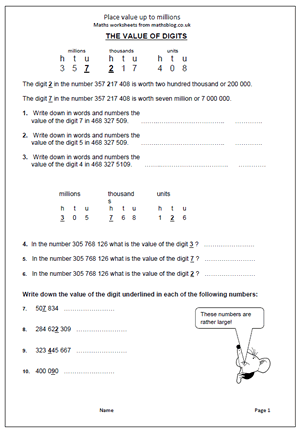Children are expected to work with even larger numbers in Year 5, including millions. Many will have difficulties in reading and writing such large numbers and will need a lot of help to appreciate the place value of each digit.

The easiest way to read  large numbers is to think of them as blocks of three, starting on the right.

Each block of three is hundreds, tens and units, which keep repeating themselves.

The first block of three are HUNDREDS, TENS AND UNITS of ones or units.

So  456 is four hundred and fifty six (units).

The second block of three are HUNDREDS, TENS AND UNITS of thousands.

So 456 456 is four hundred and fifty six thousand four hundred and fifty six.

This page takes a closer look at reading large numbers and finding the value of various digits.

Place value up to millions

## Subtraction in columns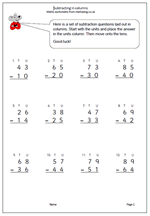It is suggested in the new Maths Programme of Study that children in Year 2 should get used to recording addition nand subtraction in columns, both to support place value and to prepare for formal written methods with larger numbers in later years.

Here we have a subtraction worksheet laid out in columns. There is no ‘borrowing’ so it is a simple matter of subtracting the units and subtracting the tens.

Subtract 2-digit numbers in columns

## Learning the ten times table in Year 2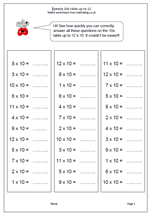Children are introduced to multiplication tables in Year 2 (6/7 yrs old) and should be fluent with the two times, five times and ten times tables by the end of the year. The ten times table is obviously the easiest to learn off by heart, but it can also be used to illustrate place value. The 5 in 50 has ten times the value of the 5 in 5.

Don’t ever suggest that all that needs to be done to multiply by ten is ‘add a nought’. This leads to real problems in later years when multiplying by decimals. Far better to say that the digit moves one place to the left and a zero is put in the units as a place holder.

Speedy 10x table

## Counting in multiples of 6, 7, 8 and 9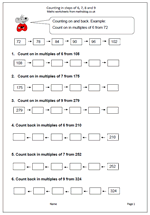A new target in the Maths Primary Framework for Year 4 is to count up in steps of 6, 7, and 9. This might sound easy and if the numbers are low there should not be a problem. However, counting up and down in steps of 6 or 7 starting from larger numbers can be tricky and might well involve a great deal of finger counting. Don’t be surprised at this as there is a lot of mathematical knowledge needed to successfully and quickly count up 7 from a number such as 196. It can be done by counting in single digits, or counting 4 to 200 and then 3, or even adding 10 and subtracting 3.

Counting in steps of 6,7,8 and 9

## Five times table in Year 2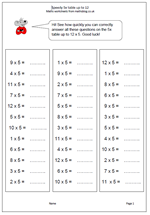Learning the five times table has now been placed firmly in the Year 2 Programme of Study and will prove quite a challenge for many children who find counting up to 100 hard, let alone reciting the 5 times table. To make it even more of a challenge the table now has to be learned up to 12 times 5 rather than just 10 times 5. There is a danger with this that children will learn the table ‘parrot fashion’ with little idea of what it means or how to use the knowledge.

There is, of course, a rhythm and pattern to the five times table, as alternate answers end in zero or 5. This makes it one of the easiest times tables to learn. My latest worksheet can be used as a timed practice, with three sets of 12 questions on the page.

Speedy 5x table

## Two times table up to 12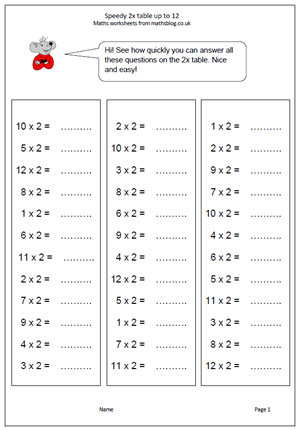In the new Maths Programme of Study children will be taught to recall and use the two times table up to twelve times two. There are many ways that times tables can be learned, but the end product will be the same; children will know the facts off by heart and be able to answer instantly. Note: knowing the two times table is not the same as being able to count up in twos. It is no good if a child has to start counting to work out what 9 x 2 is as it will take too long.

This page extends our current times table worksheets found in the Four Rules section and can be found in the Year 2 Calculating section.

Speedy 2x table up to 12

## New Maths Curriculum: Year 2 Statistics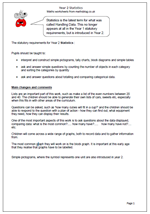Statistics is the latest term for what was called Handling Data. This no longer appears at all in the Year 1 statutory requirements, but is introduced in Year 2.

The statutory requirements for Year 2 Statistics :

Pupils should be taught to:

•    interpret and construct simple pictograms, tally charts, block diagrams and simple tables

•    ask and answer simple questions by counting the number of objects in each category and sorting the categories by quantity

Lists are an important part of this work, such as make a list of the even numbers between 20 and 40. The children should be able to generate their own lists of cars, sweets etc, especially when this fits in with other areas of the curriculum.

Questions can be asked, such as ‘how many cubes will fit in a cup?’ and the children should be able to respond to the question with a plan of action – how they can find out, what equipment they need, how they can display their results.

One of the most important aspects of this work is to ask questions about the data displayed, comparing data: what is the most common?….. how many have?…..    how many have not?…. etc.

Children will come across a wide range of graphs, both to record data and to gather information from.

The most common graph they will work on is the block graph. It is important at this early age that they realise that graphs have to be labelled.

Simple pictograms, where the symbol represents one unit are also introduced in year 2.

New Maths Curriculum: Statistics

## New Maths Curriculum: Year 2 Geometry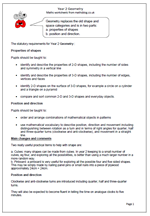Geometry replaces the old shape and space categories and is in two parts:

a. properties of shapes             b. position and direction.

The statutory requirements for Year 2 Geometry :

Properties of shapes

Pupils should be taught to:

•    identify and describe the properties of 2-D shapes, including the number of sides and symmetry in a vertical line

•    identify and describe the properties of 3-D shapes, including the number of edges, vertices and faces

•    identify 2-D shapes on the surface of 3-D shapes, for example a circle on a cylinder and a triangle on a pyramid

•    compare and sort common 2-D and 3-D shapes and everyday objects.

Position and direction

Pupils should be taught to:

•    order and arrange combinations of mathematical objects in patterns

•    use mathematical vocabulary to describe position, direction and movement including distinguishing between rotation as a turn and in terms of right angles for quarter, half and three-quarter turns (clockwise and anti-clockwise), and movement in a straight line.

Two really useful practical items to help with shape are:

a. Cubes: many shapes can be made from cubes. In year 2 keeping to a small number of cubes, eg four, and exploring all the possibilities, is better than using a much larger number in a more random way.
b. Pinboard: a pinboard is very useful for exploring all the possible four and five sided shapes. This may be simply made by nailing panel pins or small nails into a piece of plywood approximately 24cm × 24cm.

Position and direction
Clockwise and anti-clockwise turns are introduced including quarter, half and three-quarter turns.
They will also be expected to become fluent in telling the time on analogue clocks to five minutes.

New Maths Curriculum: Year 2 Geometry

## New Maths Curriculum: Year 2 Measurement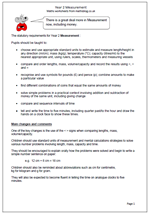The statutory requirements for Year 2 Measurement :

Pupils should be taught to:

•    choose and use appropriate standard units to estimate and measure length/height in any direction (m/cm); mass (kg/g); temperature (°C); capacity (litres/ml) to the nearest appropriate unit, using rulers, scales, thermometers and measuring vessels

•    compare and order lengths, mass, volume/capacity and record the results using >, < and =

•    recognise and use symbols for pounds (£) and pence (p); combine amounts to make a particular value

•    find different combinations of coins that equal the same amounts of money

•    solve simple problems in a practical context involving addition and subtraction of money of the same unit, including giving change

•    compare and sequence intervals of time

•    tell and write the time to five minutes, including quarter past/to the hour and draw the hands on a clock face to show these times.

One of the key changes is the use of the < > signs when comparing lengths, mass, volume/capacity.

Children should use standard units of measurement and mental calculations strategies to solve various number problems involving length, mass, capacity and time.

They should be encouraged to explain orally how the problems were solved and begin to write a simple number sentence on paper:

e.g.  12 cm + 6 cm = 18 cm

Children should also be reminded about abbreviations such as cm for centimetre,
kg for kilogram and g for gram.

They will also be expected to become fluent in telling the time on analogue clocks to five minutes.

New Maths Curriculum: Year 2 Measurement

## New Maths Curriculum: Year 2 Fractions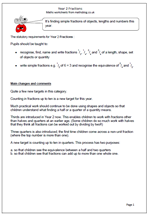Fractions are seen as a completely separate area now, rather than being within Number or Division and Fractions.

The statutory requirements for Year 2 Fractions :

Pupils should be taught to:

•    recognise, find, name and write fractions 1/3, 1/4, 2/4 and 3/4 of a length, shape, set of objects or quantity

•    write simple fractions e.g. 1/2 of 6 = 3 and recognise the equivalence of 2/4 and 1/2.

Quite a few new targets in this category.
Counting in fractions up to ten is a new target for this year.
Much practical work should continue to be done using shapes and objects so that children understand what finding a half or a quarter of a quantity means.

Thirds are introduced in Year 2 now. This enables children to work with fractions other than halves and quarters at an earlier age. (Some children do so much work with halves that they think all fractions can be worked out by dividing by two!!)

Three quarters is also introduced; the first time children come across a non-unit fraction (where the top number is more than one).

A new target is counting up to ten in quarters. This process has two purposes:

a. so that children see the equivalence between a half and two quarters
b. so that children see that fractions can add up to more than one whole one.

New Maths Curriculum: Fractions